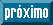## Indicadores

•Citado por SciELO
•Acessos

## Links relacionados

•Similares em SciELO

## versão impressa ISSN 2007-1132

#### Resumo

HERNANDEZ RAMOS, Jonathan et al. Generalized height-diameter equations for Pinus teocote Schlecht. & Cham. in the state of Hidalgo. Rev. mex. de cienc. forestales [online]. 2015, vol.6, n.31, pp.08-21. ISSN 2007-1132.

The height-diameter relationship is important in forest inventories to determine the stock and growth models. However, measuring the height entails greater difficulty and cost, so an option is to do it by means of a subsample, for which the use of equations is convenient. The objective of this study was to select a generalized best fit equation to estimate the relationship of total height as a function of normal diameter (h-d) for pure pine stands of Pinus teocote in the eastern area of the state of Hidalgo. A total of 1 985 pairs of h-d data were used; 1 802 pairs were utilized to adjust the 13 equations by means of the MODEL procedure and the SUR technique, and 183 pairs, to validate them. The equations were selected based on a goodness-of-fit test with Mean Square Error and R2adjusted. The normality of the data, the heterogeneity of the variances and the homogeneity of the residuals were verified. The Gadow and Hui equation displayed the best fit with R2adjusted = 0.9396 and Root MSE = 1.628. The values of the Shapiro-Wilk test (0.982776),the Durbin-Watson test (1.831) and the Breusch-Pagan test (2.71) and the absolute bias (0.013) validate the equation. No significant differences between the predicted and the real values were found (t test at 95 %). With this model, it is possible to estimate the total height of P. teocote on the basis of the normal diameter, the square diameter and the mean height of the trees at the site.

Palavras-chave : Total height; normal diameter; generalized equation; nonlinear models; Pinus teocote Schlecht. & Cham.; tand.

· resumo em Espanhol     · texto em Espanhol     · Espanhol (pdf )Todo o conteúdo deste periódico, exceto onde está identificado, está licenciado sob uma Licença Creative Commons